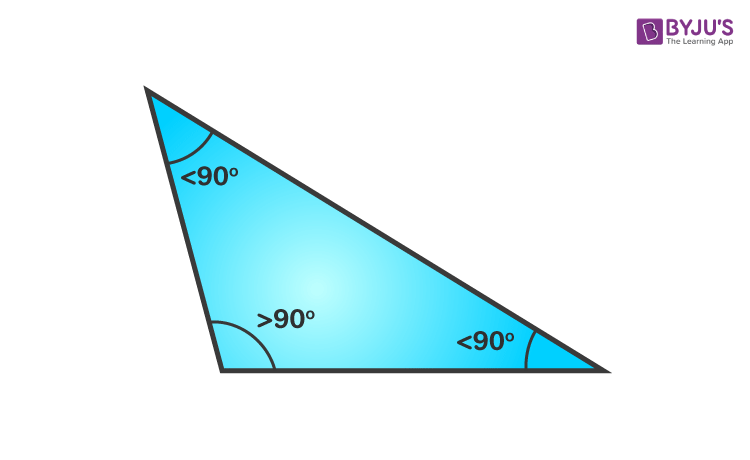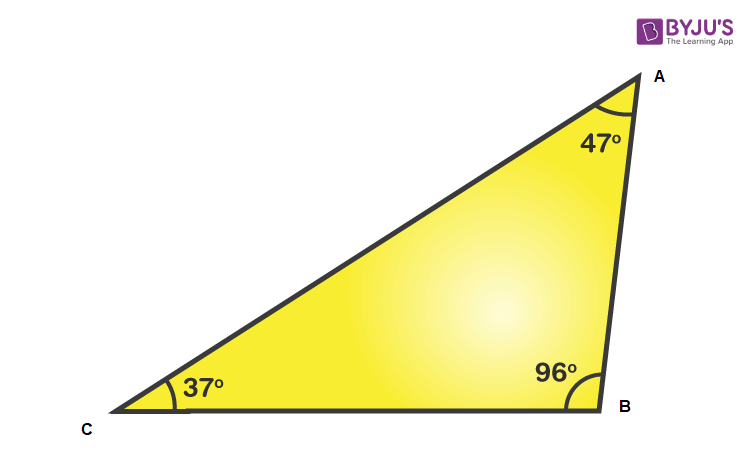# Obtuse Angled Triangle

A triangle is a closed two-dimensional plane figure with three sides and three angles. Based on the sides and the interior angles of a triangle, different types of triangles are obtained and the obtuse-angled triangle is one among them. If one of the interior angles of the triangle is obtuse (i.e. more than 90°), then the triangle is called the obtuse-angled triangle.

The obtuse angle in the triangle can be any one of the three angles and the remaining two angles are acute. This triangle is also said as an obtuse triangle. Acute and right triangles are the other two triangles apart from obtuse, which are based on the angles. But acute and obtuse are the best examples of a scalene triangle.

## Obtuse Angled Triangle Definition

A triangle whose any one of the angles is an obtuse angle or more than 90°, then it is called an obtuse-angled triangle or obtuse triangle. The sum of the interior angles of the obtuse triangle is equal to 180° only. This means the angle sum property for any triangle remains the same.

Thus, if one angle is obtuse or more than 90°, then the other two angles are definitely acute or both the angles are less than 90°.The obtuse angle triangle properties are different from other triangles. Look at the table below for the same:

 Triangle type Obtuse Acute Right Difference Any One angle more than 90° All angles less than 90° One angle equal to 90°

### Obtuse Angle

Since we are learning here about an obtuse-angled triangle, thus it is necessary to know, what actually an obtuse angle is?

There are basically three types of angles formed when we join any two-line segments end to end. They are:

• Acute angle
• Right angle
• Obtuse angle

An acute angle is formed when two line segments are joined in such a way, that the angle between them is less than 90°. The triangle resulting from this angle is called an acute angle triangle.

A right angle is formed when one line segment is exactly perpendicular to another line segment at the joining points.

Now, when we speak about the obtuse angle, two line segments are joined in such a way that the angle between them is more than 90°. And when we join the other two open ends of the line segments, then it results in an obtuse-angled triangle.

## Obtuse Angled Triangle Formula

The formula for the area and perimeter of an obtuse triangle is similar to the formula for any other triangle.

Hence, the area of the triangle is given by:

Area = 1/2 × b × h

where b is the base and h is the height of the triangle.

Or

$$\begin{array}{l}A=\sqrt{s(s-a)(s-b)(s-c)}sq.units\end{array}$$

Where s = (a+b+c)/2 (s= semiperimeter)

where a, b and c are the length of the sides of the triangle.

The perimeter of the triangle is always equal to the sum of the sides of the triangle. Hence, if a, b and c are the sides of the obtuse triangle, then the perimeter is given by;

Perimeter = a+b+c

### How do you know if a triangle is obtuse

If any two angles of a triangle are given, it can be easily determined whether the triangle is an obtuse triangle or not. But how to determine this, when the three sides of the triangle are known? We have inequality in the lines of Pythagorean identity to test this.

The triangle is an obtuse triangle if the sum of the squares of the smaller sides is less than the square of the largest side.

Let a, b and c are the lengths of the sides of triangle ABC and c is the largest side, then the triangle is obtuse if

a2 + b2 < c2

### Obtuse Angled Triangle Properties

1. The sum of the two angles other than the obtuse angle is less than 90°.
2. The side opposite the obtuse angle is the longest side of the triangle.
3. An obtuse triangle will have one and only one obtuse angle. The other two angles are acute angles.
4. The points of concurrency, the Circumcenter and the Orthocenter lie outside of an obtuse triangle, while Centroid and Incenter lie inside the triangle.

### Example

Triangle ABC is a perfect example to study the triangle type – Obtuse.• In triangle ABC, interior angle ACB =37°, which is less than 90°, so it’s an acute angle.
• Interior angle ABC = 96°, which is more than 90° so, it’s an obtuse angle.
• Interior angle BAC=47°, which is less than 90°, so it’s an acute angle.
• As this triangle, ABC has one angle (ABC=96°) more than 90°, so this triangle is obtuse.

### Video Lesson on Types of TrianglesSubscribe to BYJU’S – The Learning App to know all about Maths related topics and also watch interactive videos to learn with ease.

Test your knowledge on Obtuse Angled Triangle